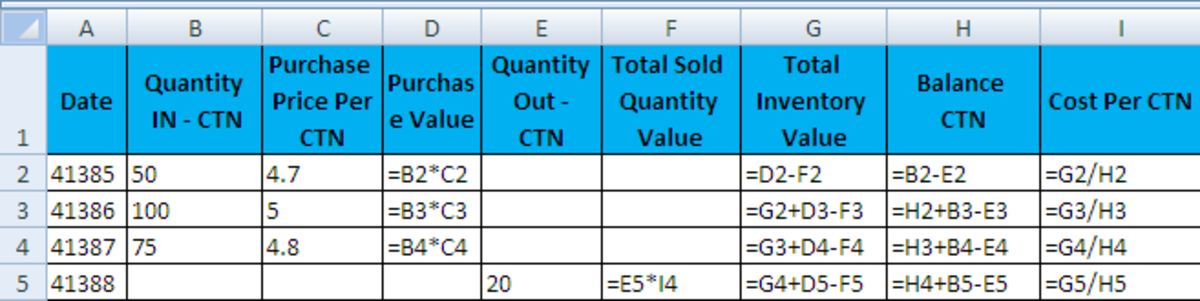# How to Calculate Weighted Average Cost of Inventory Using Microsoft Office Excel

## Why is it important to calculate Weighted Average Cost (AVCO)

Everyone knows that profit margin is the difference between Cost & Revenue. The revenue is the price which I get from or charge to my customer, but the issue is how to calculate my cost per carton or piece..etc to be able to calculate my profit Margin per unit.

Here we will discuss many scenarios.

## Scenario 1 - Using One Unit of Measure

Here we will discuss when we purchase the Item measured by one unit & we resell it using same unit.

It is the simplest scenario; let us say that we did the following:

1. On 21-4-2013, we purchased 50 Cartons (CTN) for \$4.7 per CTN.
2. On 22-4-2013, we purchased 100 CTN for \$5 per CTN.
3. On 23-4-2013, we purchased 75 CTN for \$4.8 per CTN.
4. On 24-4-2013, we sold 20 CTN for \$6 per CTN.

### Questions:

1. How much profit Margin per carton (unit) did we earn?
2. How much does the sold quantity cost?
3. How much does remaining stock (Inventory or quantity on hand QOH) cost?

## The formulas used

Go to Excel and add below columns:

1. In cell A1, type (Date).
2. In cell B1, type (Quantity IN – CTN).
3. In cell C1, type (Purchase Price Per CTN).
4. In cell D1, type (Purchase Value).
5. Now fill the purchased quantities & the prices.
6. In Cell D2, type =B2*C2 so you get \$235 which come from multiplying 50 cartons purchased by \$4.7 purchase price.
7. Copy D2 cell & paste it in cell D3 & D4 or easily select the cells from D2 till D4 & hit Ctrl+D, this will duplicate the formula which you typed in D2 (Note: Use Ctrl+D to duplicate any upper cell, it is a very helpful shortcut).
8. In cell A5, type total.
9. In cell B5, type =SUM(B2:B4) , so you get 225 which is the total number of cartons purchased (50+100+75).
10. Copy B5 & paste in cell D5; you get total of your purchases in dollar value (\$235+\$500+\$360).
11. Chose any cell like D8 and divide total purchase value D5 by number of cartons purchased B5, type this formula =D5/B5
12. Now you get Weighted Average Cost of one CTN = \$4.87.

Now lets us answer the questions

1. Profit Margin per Unit = Selling Price per Unit - Weighted Average Cost per Unit (=\$6-\$4.87= \$1.13)
2. The Sold Quantity cost = 20 Cartons * \$4.87 = \$97.4
3. Remaining or available stock (Inventory) cost = Total cost of purchases - Sold Quantity cost (= \$1095-\$97.4,=\$997.6)

## Weighted Average Cost of Inventory

Scroll to Continue

## More Excel Hubs

The previous answer is correct, but it is not flexible for adding more transactions and doing more calculations that are complicated. You can see that we answered all our questions by doing some extra calculations.

So, I suggest that you should use the below table.

As you can see that using this table will give you below mentioned details:

1. Available Quantity on hand (QOH) or (Balance of cartons) after every transaction, check column (H), after first purchase, it was 50 CTN then increased by 100 CTN to be 150 CTN, and so on.
2. Total Cost of Inventory after every transaction, check column (G), it was \$235 then increased by \$500 to be \$735, and so on.
3. Cost per Unit after every transaction, this in column (I), it was 4.7 or 235/50 then increased to 4.9 or 735/150 and so on.
4. Total cost of every sales transaction, you can get it from column (F).
5. Total cost of every purchase transaction, look at column (D), it was 235 or 50*4.7, then 500 or 100*5, and so on. Note: do not use this column when sales happen.

Note: If you want to fill more transactions, just copy the formulas to below (remember you can use Ctrl+D shortcut).

## The formulas usedCalculating Weighted Average Cost of inventory - The FormulasOriginal by Taleb80